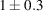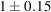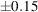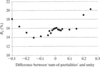International
Tables for
Crystallography
Volume F
Crystallography of biological macromolecules
Edited by M. G. Rossmann and E. Arnold

International Tables for Crystallography (2006). Vol. F. ch. 11.5, p. 239   | 1 | 2 |

## Section 11.5.7.2. R factor as a function of sum-of-partialities' (method 1)C. G. van Beek,a R. Bolotovskya§ and M. G. Rossmanna*

aDepartment of Biological Sciences, Purdue University, West Lafayette, IN 47907-1392, USA
Correspondence e-mail:  mgr@indiana.bio.purdue.edu

#### 11.5.7.2. R factor as a function of sum-of-partialities' (method 1)| top | pdf |

In order to determine the limits of tolerance that can be permitted when method 1is used, the R factor was examined as a function of the sum-of-partialities for the ϕX174 procapsid data (Fig. 11.5.7.4). Reflections with sum-of-partialities ofwere used. The R factor changes sharply when the sum-of-partialities is outside. Hence,were acceptable limits of tolerance for this data set.Figure 11.5.7.4 | top | pdf |R factor as a function of the difference of calculated `sum-of-partialities' and unity for the estimates of full reflections when method 1is used for the scaling and averaging of a ϕX174 data set (Dokland et al., 1997).